# Deep Learning Course

You can find here slides, recordings, and a virtual machine for François Fleuret's deep-learning courses 14x050 of the University of Geneva, Switzerland.

This course is a thorough introduction to deep-learning, with examples in the PyTorch framework:

• machine learning objectives and main challenges,
• tensor operations,
• deep-learning specific techniques,
• generative, recurrent, attention models.

You can check the pre-requisites.

This course was developped initialy at the Idiap Research Institute in 2018, and taught as EE-559 at École Polytechnique Fédérale de Lausanne until 2022. The notes for the handouts were added with the help of Olivier Canévet.

Thanks to Adam Paszke, Jean-Baptiste Cordonnier, Alexandre Nanchen, Xavier Glorot, Andreas Steiner, Matus Telgarsky, Diederik Kingma, Nikolaos Pappas, Soumith Chintala, and Shaojie Bai for their answers or comments.

# Lecture materials

The slide pdfs are the ones I use for the lectures. They are in landscape format with overlays to facilitate the presentation. The handout pdfs are compiled without these fancy effects in portrait orientation, with additional notes. The screencasts are available both as in-browser streaming or downloadable mp4 files.

You can get archives with all the pdf files (1097 slides):

and subtitles for the screencasts generated automaticallly with OpenAI's Whisper:

or the individual lectures:

• 1. Introduction. (90 slides, 1h57min videos)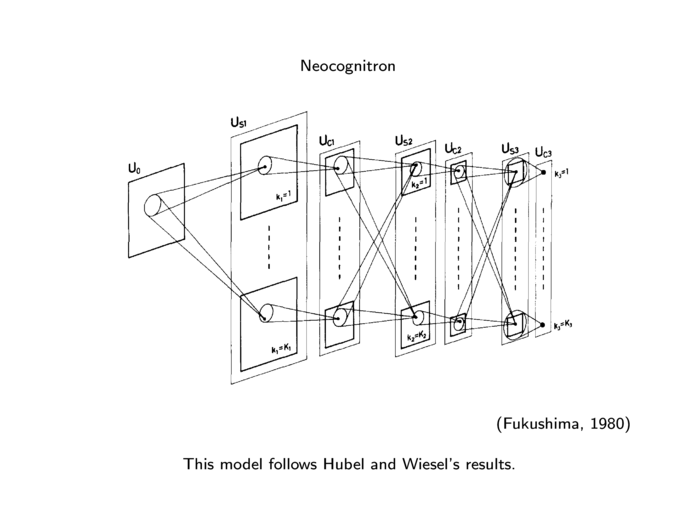1.1. From neural networks to deep learning. (18 slides, 26min video) handout (slides), stream (mp4). 1.2. Current applications and success. (25 slides, 29min video) handout (slides), stream (mp4). 1.3. What is really happening? (10 slides, 11min video) handout (slides), stream (mp4). 1.4. Tensor basics and linear regression. (13 slides, 21min video) handout (slides), stream (mp4). 1.5. High dimension tensors. (20 slides, 25min video) handout (slides), stream (mp4). 1.6. Tensor internals. (4 slides, 6min video) handout (slides), stream (mp4).
• 2. Machine learning fundamentals. (72 slides, 1h44min videos)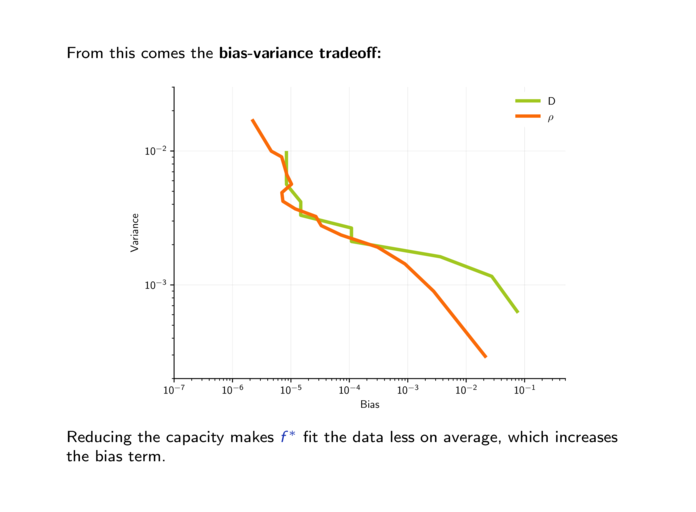2.1. Loss and risk. (12 slides, 20min video) handout (slides), stream (mp4). 2.2. Over and under fitting. (25 slides, 36min video) handout (slides), stream (mp4). 2.3. Bias-variance dilemma. (10 slides, 18min video) handout (slides), stream (mp4). 2.4. Proper evaluation protocols. (6 slides, 11min video) handout (slides), stream (mp4). 2.5. Basic clusterings and embeddings. (19 slides, 19min video) handout (slides), stream (mp4).
• 3. Multi-layer perceptron and back-propagation. (68 slides, 1h54min videos)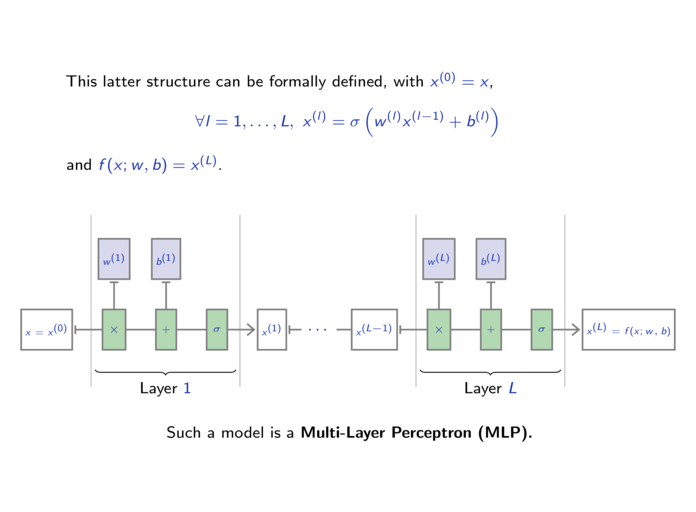3.1. The perceptron. (16 slides, 28min video) handout (slides), stream (mp4). 3.2. Probabilistic view of a linear classifier. (8 slides, 14min video) handout (slides), stream (mp4). 3.3. Linear separability and feature design. (10 slides, 17min video) handout (slides), stream (mp4). 3.4. Multi-Layer Perceptrons. (10 slides, 11min video) handout (slides), stream (mp4). 3.5. Gradient descent. (13 slides, 24min video) handout (slides), stream (mp4). 3.6. Back-propagation. (11 slides, 20min video) handout (slides), stream (mp4).
• 4. Graphs of operators, autograd, and convolutional layers. (86 slides, 1h36min videos)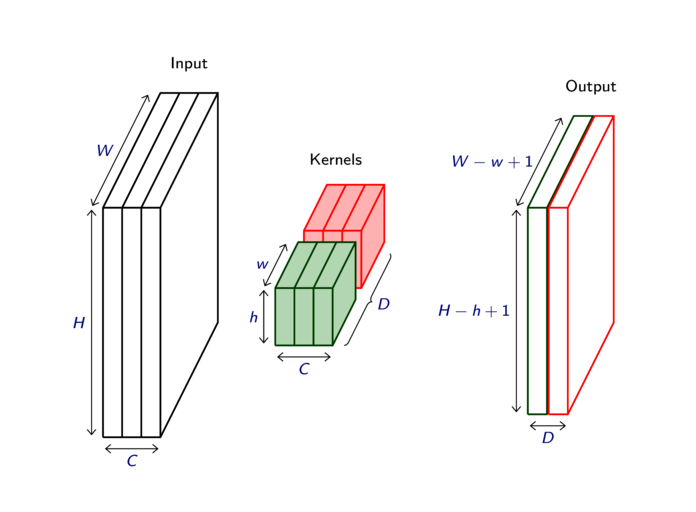4.1. DAG networks. (11 slides, 21min video) handout (slides), stream (mp4). 4.2. Autograd. (20 slides, 22min video) handout (slides), stream (mp4). 4.3. PyTorch modules and batch processing. (15 slides, 15min video) handout (slides), stream (mp4). 4.4. Convolutions. (23 slides, 23min video) handout (slides), stream (mp4). 4.5. Pooling. (7 slides, 5min video) handout (slides), stream (mp4). 4.6. Writing a PyTorch module. (10 slides, 10min video) handout (slides), stream (mp4).
• 5. Initialization and optimization. (81 slides, 1h42min videos)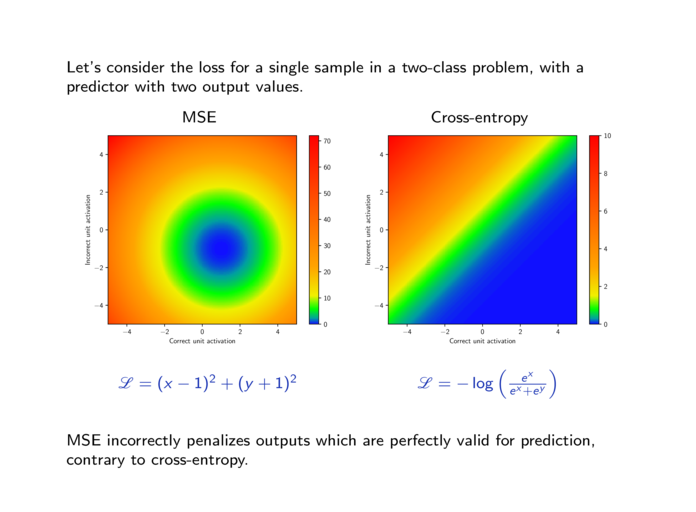5.1. Cross-entropy loss. (9 slides, 17min video) handout (slides), stream (mp4). 5.2. Stochastic gradient descent. (17 slides, 26min video) handout (slides), stream (mp4). 5.3. PyTorch optimizers. (8 slides, 6min video) handout (slides), stream (mp4). 5.4. L2 and L1 penalties. (11 slides, 13min video) handout (slides), stream (mp4). 5.5. Parameter initialization. (20 slides, 19min video) handout (slides), stream (mp4). 5.6. Architecture choice and training protocol. (9 slides, 13min video) handout (slides), stream (mp4). 5.7. Writing an autograd function. (7 slides, 8min video) handout (slides), stream (mp4).
• 6. Going deeper. (86 slides, 1h39min videos)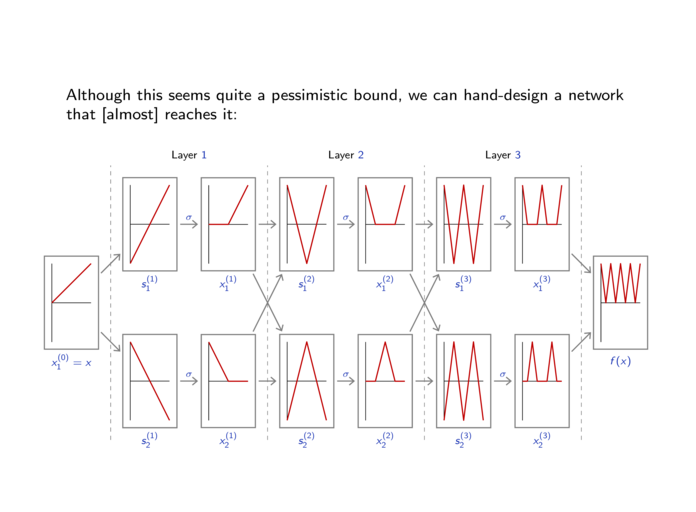6.1. Benefits of depth. (12 slides, 24min video) handout (slides), stream (mp4). 6.2. Rectifiers. (7 slides, 4min video) handout (slides), stream (mp4). 6.3. Dropout. (11 slides, 13min video) handout (slides), stream (mp4). 6.4. Batch normalization. (16 slides, 19min video) handout (slides), stream (mp4). 6.5. Residual networks. (21 slides, 22min video) handout (slides), stream (mp4). 6.6. Using GPUs. (19 slides, 18min video) handout (slides), stream (mp4).
• 7. Autoencoders. (93 slides, 1h22min videos)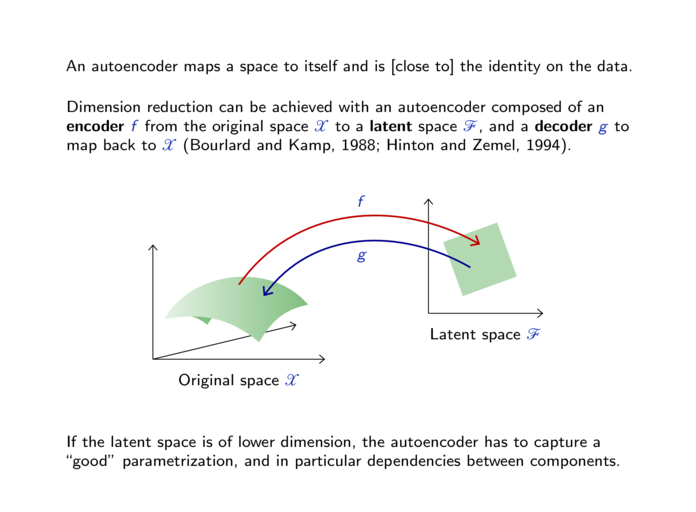7.1. Transposed convolutions. (14 slides, 14min video) handout (slides), stream (mp4). 7.2. Deep Autoencoders. (26 slides, 16min video) handout (slides), stream (mp4). 7.3. Denoising autoencoders. (38 slides, 33min video) handout (slides), stream (mp4). 7.4. Variational autoencoders. (15 slides, 19min video) handout (slides), stream (mp4).
• 8. Computer vision. (88 slides, 1h49min videos)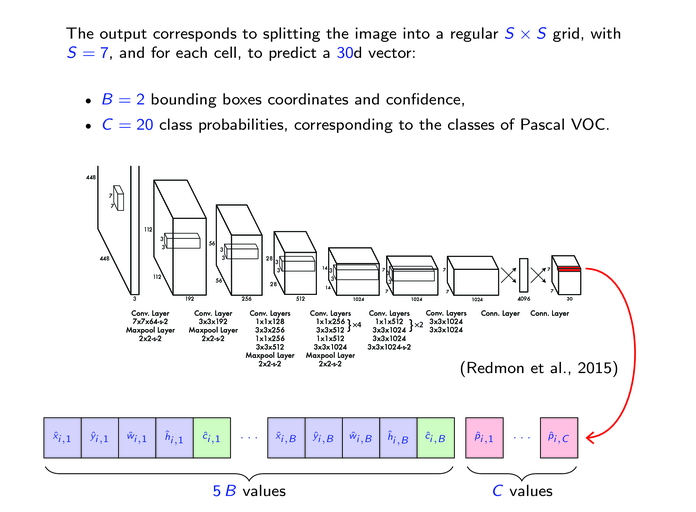8.1. Computer vision tasks. (14 slides, 20min video) handout (slides), stream (mp4). 8.2. Networks for image classification. (36 slides, 44min video) handout (slides), stream (mp4). 8.3. Networks for object detection. (15 slides, 21min video) handout (slides), stream (mp4). 8.4. Networks for semantic segmentation. (10 slides, 11min video) handout (slides), stream (mp4). 8.5. DataLoader and neuro-surgery. (13 slides, 13min video) handout (slides), stream (mp4).
• 9. Under the hood. (92 slides, 1h22min videos)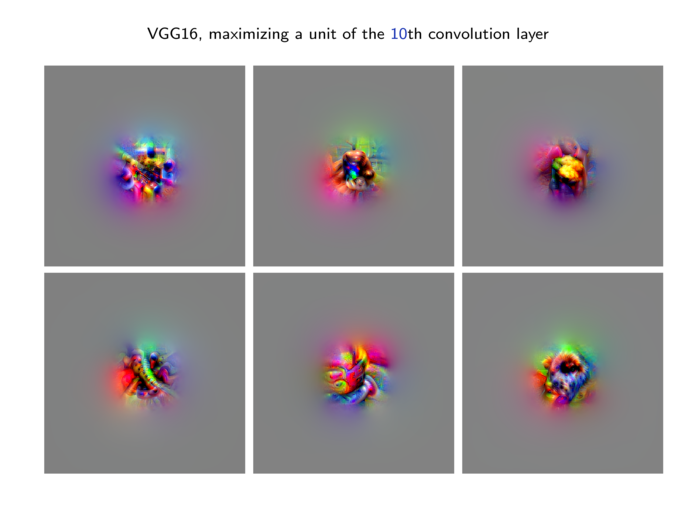9.1. Looking at parameters. (13 slides, 10min video) handout (slides), stream (mp4). 9.2. Looking at activations. (20 slides, 23min video) handout (slides), stream (mp4). 9.3. Visualizing the processing in the input. (34 slides, 23min video) handout (slides), stream (mp4). 9.4. Optimizing inputs. (25 slides, 25min video) handout (slides), stream (mp4).
• 10. Autoregression and Normalizing Flows. (84 slides, 1h27min videos)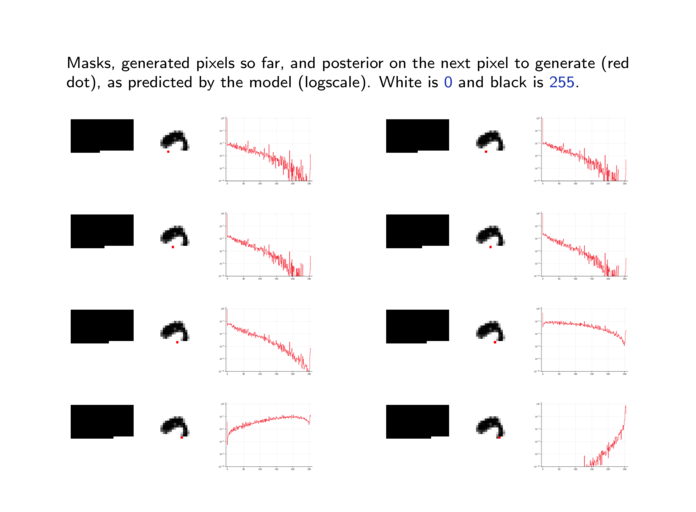10.1. Auto-regression. (25 slides, 28min video) handout (slides), stream (mp4). 10.2. Causal convolutions. (25 slides, 22min video) handout (slides), stream (mp4). 10.3. Non-volume preserving networks. (34 slides, 37min video) handout (slides), stream (mp4).
• 11. Generative Adversarial Networks. (91 slides, 1h22min videos)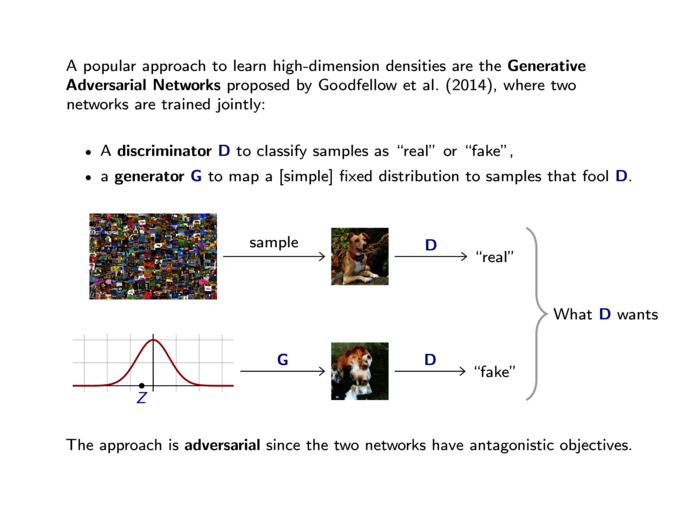11.1. Generative Adversarial Networks. (33 slides, 30min video) handout (slides), stream (mp4). 11.2. Wasserstein GAN. (20 slides, 24min video) handout (slides), stream (mp4). 11.3. Conditional GAN and image translation. (29 slides, 20min video) handout (slides), stream (mp4). 11.4. Model persistence and checkpoints. (9 slides, 8min video) handout (slides), stream (mp4).
• 12. Recurrent models and NLP. (73 slides, 1h18min videos)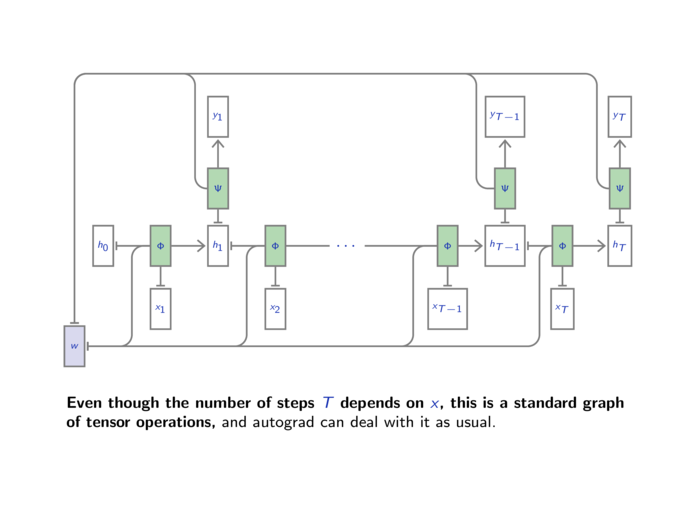12.1. Recurrent Neural Networks. (24 slides, 23min video) handout (slides), stream (mp4). 12.2. LSTM and GRU. (17 slides, 14min video) handout (slides), stream (mp4). 12.3. Word embeddings and translation. (32 slides, 41min video) handout (slides), stream (mp4).
• 13. Attention models. (the screencasts are not up-to-date, check the slides! – 93 slides, 1h25min videos)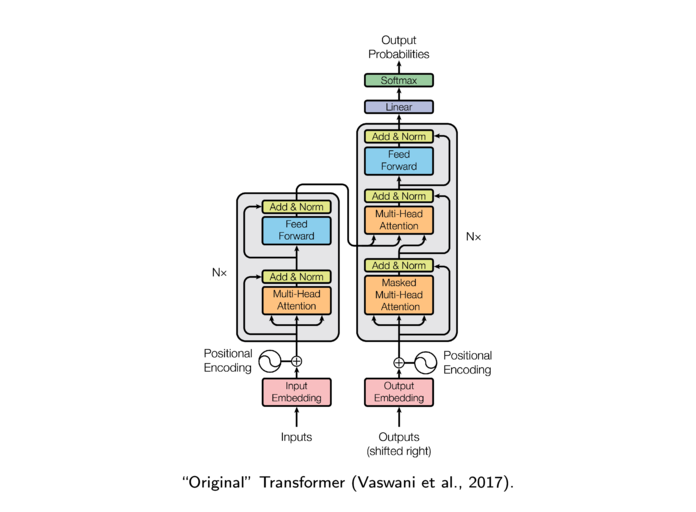13.1. Attention for Memory and Sequence Translation. (21 slides, 21min video) handout (slides), stream (mp4). 13.2. Attention Mechanisms. (30 slides, 30min video) handout (slides), stream (mp4). 13.3. Transformer Networks. (42 slides, 34min video) handout (slides), stream (mp4).

# Information

## Pre-requisites

• Linear algebra (vectors, matrices, Euclidean spaces),
• differential calculus (Jacobian, Hessian, chain rule),
• Python programming,
• basics in probabilities and statistics (discrete and continuous distributions, law of large numbers, conditional probabilities, Bayes, PCA),
• basics in optimization (notion of minima, gradient descent),
• basics in algorithmic (computational costs),
• basics in signal processing (Fourier transform, wavelets).

## Documentation

You may have to look at the Python, Jupyter notebook, and PyTorch documentations at

## Practical session prologue

Helper Python prologue for the practical sessions: dlc_practical_prologue.py

### Argument parsing

This prologue parses command-line arguments as follows

```usage: dummy.py [-h] [--full] [--tiny] [--seed SEED]
[--cifar] [--data_dir DATA_DIR]

DLC prologue file for practical sessions.

optional arguments:
-h, --help           show this help message and exit
--full               Use the full set, can take ages (default
False)
--tiny               Use a very small set for quick checks
(default False)
--seed SEED          Random seed (default 0, < 0 is no seeding)
--cifar              Use the CIFAR data-set and not MNIST
(default False)
--data_dir DATA_DIR  Where are the PyTorch data located (default
\$PYTORCH_DATA_DIR or './data')
```

The prologue provides the function

```load_data(cifar = None, one_hot_labels = False, normalize = False, flatten = True)
```

which downloads the data when required, reshapes the images to 1d vectors if flatten is True, and narrows to a small subset of samples if --full is not selected.

It returns a tuple of four tensors: train_data, train_target, test_data, and test_target.

If cifar is True, the data-base used is CIFAR10, if it is False, MNIST is used, if it is None, the argument --cifar is taken into account.

If one_hot_labels is True, the targets are converted to 2d torch.Tensor with as many columns as there are classes, and -1 everywhere except the coefficients [n, y_n], equal to 1.

If normalize is True, the data tensors are normalized according to the mean and variance of the training one.

If flatten is True, the data tensors are flattened into 2d tensors of dimension N × D, discarding the image structure of the samples. Otherwise they are 4d tensors of dimension N × C × H × W.

### Minimal example

```import dlc_practical_prologue as prologue

train_input, train_target, test_input, test_target = prologue.load_data()

print('train_input', train_input.size(), 'train_target', train_target.size())
print('test_input', test_input.size(), 'test_target', test_target.size())
```

prints

```* Using MNIST
** Reduce the data-set (use --full for the full thing)
** Use 1000 train and 1000 test samples
train_input torch.Size([1000, 784]) train_target torch.Size()
test_input torch.Size([1000, 784]) test_target torch.Size()
```

# Virtual Machine

A Virtual Machine (VM) is a software that simulates a complete computer. The one we provide here includes a Linux operating system and all the tools needed to use PyTorch from a web browser (e.g. Mozilla Firefox or Google Chrome).

### Installation

3. open the latter in VirtualBox with File → Import Appliance.

You should now see an entry in the list of VMs. The first time it starts, it provides a menu to choose the keyboard layout you want to use (you can force the configuration later by running the command sudo set-kbd).

If the VM does not start and VirtualBox complains that the VT-x is not enabled, you have to activate the virtualization capabilities of your CPU in the BIOS of your computer.

### Using the VM

The VM automatically starts a JupyterLab on port 8888 and exports that port to the host. This means that you can access this JupyterLab with a web browser on the machine running VirtualBox at http://localhost:8888/ and use Python notebooks, view files, start terminals, and edit source files. Typing !bye in a notebook or bye in a terminal will shutdown the VM.

You can run a terminal and a text editor from inside the Jupyter notebook for exercises that require more than the notebook itself. Source files can be executed by running in a terminal the Python command with the source file name as argument. Both can be done from the main Jupyter window with:

• New → Text File to create the source code, or selecting the file and clicking Edit to edit an existing one.
• New → Terminal to start a shell from which you can run Python.

This VM also exports an ssh port to the port 2022 on the host, which allows to log in with standard ssh clients on Linux and OSX, and with applications such as PuTTY on Windows. The default login is 'dave' and password 'dummy', same password for the root account.

### Remarks

Note that performance for computation will be very poor compared to installing PyTorch natively on your machine. In particular, the VM does not take advantage of a GPU if you have one.

Finally, please also note that this VM is configured in a convenient but highly non-secured manner, with easy to guess passwords, including for the root, and network-accessible non-protected Jupyter notebooks.

This VM is built on a Linux Debian, with miniconda, PyTorch, MNIST, CIFAR10, and many Python utility packages installed.